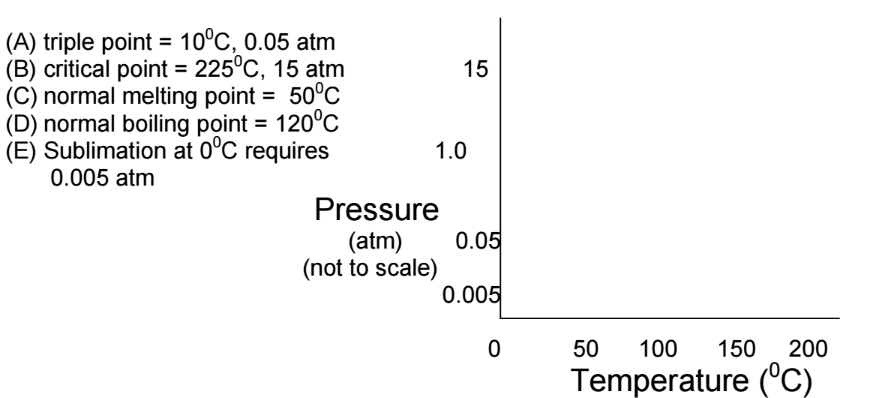# Problem: Sketch the phase diagram:Which of the following statements is true about the phase diagram above?I. The solid is denser than the liquid.II. The melting point of the solid decreases as pressure is increased.III. Line AB represents fusion.IV. As the temperature is decreased from 200°C to 0°C at 0.9 atm, two phase changes occur.V. As the pressure is increased from 0.003 atm to 14 atm at 25°C, one phase change occurs.a) I, IIb) I, IVc) II, IVd) II, Ve) IV, V

###### FREE Expert Solution
95% (370 ratings)
###### Problem Details

Sketch the phase diagram:

Which of the following statements is true about the phase diagram above?

I. The solid is denser than the liquid.

II. The melting point of the solid decreases as pressure is increased.

III. Line AB represents fusion.

IV. As the temperature is decreased from 200°C to 0°C at 0.9 atm, two phase changes occur.

V. As the pressure is increased from 0.003 atm to 14 atm at 25°C, one phase change occurs.

a) I, II

b) I, IV

c) II, IV

d) II, V

e) IV, V